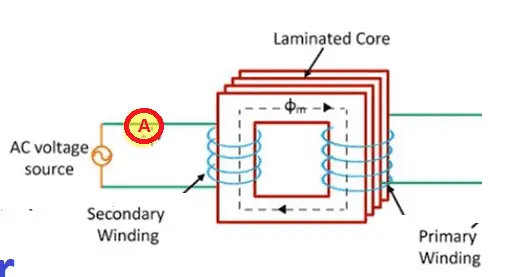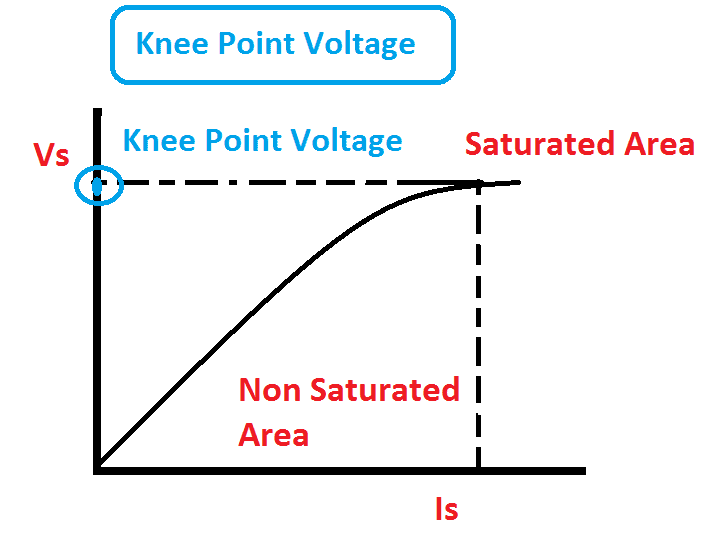# What is The Use Of Knee Point Voltage

## Knee point voltage:

Knee point voltage is nothing but a magnitude of the saturation limit of the current transformer. Each protection class CT has to be operated in its Non saturation region. So that we have to calculate the maximum allowable voltage limit, that can be applied to the secondary winding of the current transformer. As per IEC, the Knee Point Voltage of a Current Transformer is defined as the voltage at which 10 % increase in voltage of CT secondary results in 50 % increase in secondary current.

### Easy understanding of Knee Point voltage:

Consider transformer Voltage transformation ratio Es= 4.44 * flux * Current * Number of conductor. From this equation, the flux transfer to the core is directly proportional to the applied voltage and the current is directly proportional to the mmf (ampere turns.). therefore, the applied voltage in the transformer increase flux in the core, but the core has the certain limit of flux transition, beyond that limit the core opposes the flux. So that, to transformer minimum voltage the transformer’s winding takes more current due to that the core temperature will be increase. This is called core saturation. That limit is called knee point voltage.

### Knee point voltage testing procedure:

The current transformer’s secondary will be connected as shown in the figure and the primary keeps as open circuit. The input voltage to the current transformer will be increased by step by step of 10% until 120%. The secondary voltage and the current will be noted. In certain limit, applying a small voltage the transformer takes more current typically for applying 10 % of secondary voltage the transformer takes 50% of its secondary current. That voltage limits is called knee point.### Graphical representation.

Consider Vs as the current transformer secondary voltage and Is is the secondary current. using above value, please draw graph. At one point, to increase a small amount voltage the current takes higher value.### Significance of Knee Point Voltage:

Knee point Voltage is associated with the instrument safety factor. Knee point voltage will be determined in protection class CT only. (PS class- Protection Special) to know about the CT saturation level.